# Find if a character is in a range and cell in Microsoft Excel 2010

In this article, we will learn how to find if a character is in the range and cell in Microsoft Excel 2010. To find if the character is in range and cell, we use a combination of “SUMPRODUCT”, “ISNUMBER”, “SEARCH”, and “FIND” functions.

SUMPRODUCT: This function is used to add all the numbers in a range of cells.

The syntax of SUMPRODUCT formula:- =SUMPRODUCT(array1, [array2],…..)

Let’s understand with a simple exercise how we can use the SUMPRODUCT function with multiple criteria.

We have a table in Range “A2 to C16”, which contains the Agents score data. Column A contains date, Column B Agents Name, and Column C contains score. Now we need to calculate the total score of Agent 4 in cell C18. Let’s see how we can use the SUMPRODUCT function to return this value.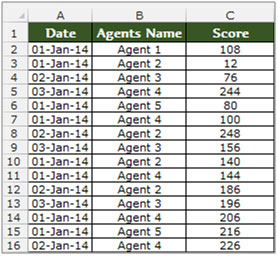• Select the cell C18, and write the formula in the cell.
• =SUMPRODUCT((B2:B16=B18)*(C2:C16))
• Press Enter on the keyboard.
• The function will return the score value of Agent 4.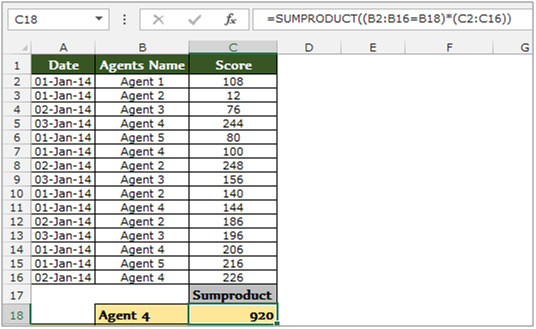ISNUMBER: This function can be used to check if a cell contains a number.

Syntax of “ISTEXT” function:       =ISTEXT (value)

Example: Cell A2 contains the text 123

=ISNUMBER (A2), function will return “TRUE”

Cell A3 contains the number “XYZ”

=ISNUMBER (A3), function will return “FALSE”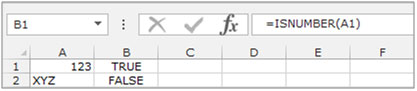SEARCH: The SEARCH function returns the starting position of a text string which it locates from within the text string.

Syntax of “SEARCH” function:      =SEARCH (find_text,within_text,[start_num])

Example: Cell A2 contains the text “Broncho Billy Anderson”

=SEARCH ("Billy", A1, 1), function will return 9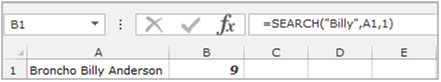FIND: This function returns the number of the character at which a specific character or text string is first found, reading left to right (not case-sensitive).

Syntax of “FIND” function:           =FIND (find_text,within_text,[start_num])

Example: Cell A1contains the text “Broncho Billy Anderson”

=FIND ("Billy", A1, 1), function will return 9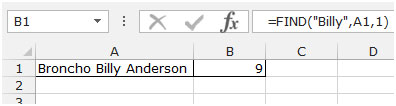Find the character in a Range

Let’s take an example to understand how we can find if a character is in a range and cell in Microsoft Excel.

The following character appears in range A2:B6. The search term we are searching for is the question mark- ?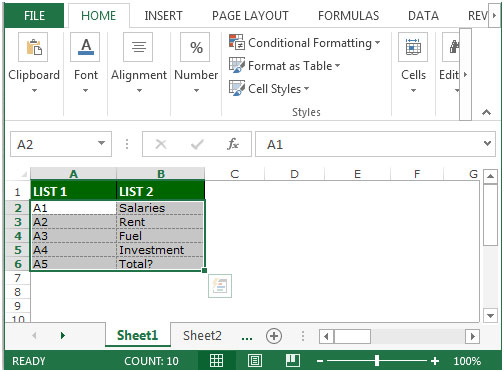Follow below given steps to search the question mark- ?

• Select the cell C2.
• Write the formula.
• =SUMPRODUCT(ISNUMBER(SEARCH("?",\$A\$2:\$A\$6))+0)>0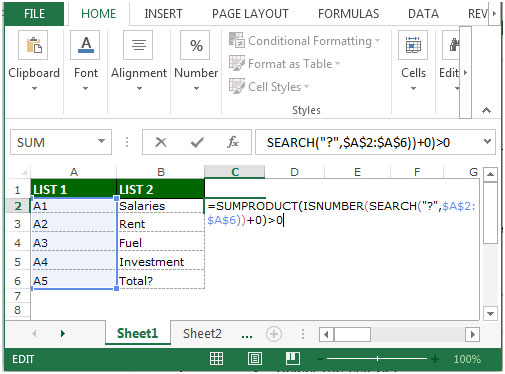• Press Enter on your keyboard.
• The function will return true.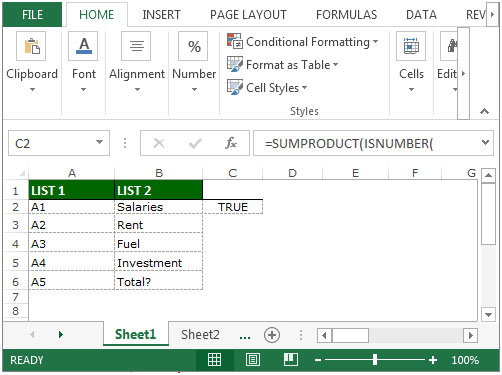This is the way you can find if a character is in a range.

Find the character in a Cell

Enter the following text in cell A1: Total?

Follow below given steps to search the question mark- ?

• Select the cell C2.
• Write the formula.
• =FIND(“?”,A1)>0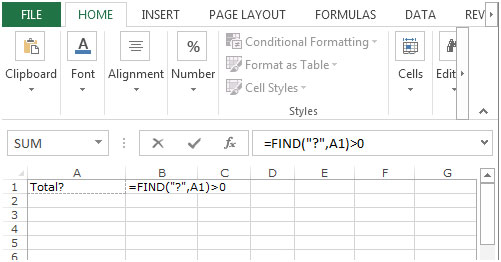• Press Enter on your keyboard.
• The function will return True.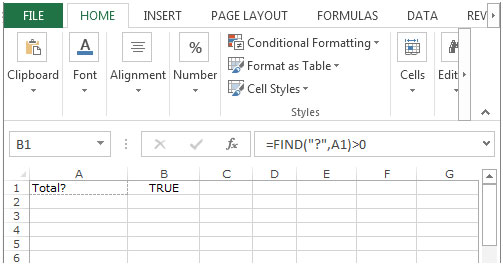This is the way by which you can find if a character is in a cell.

Terms and Conditions of use

The applications/code on this site are distributed as is and without warranties or liability. In no event shall the owner of the copyrights, or the authors of the applications/code be liable for any loss of profit, any problems or any damage resulting from the use or evaluation of the applications/code.